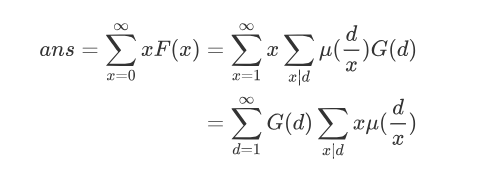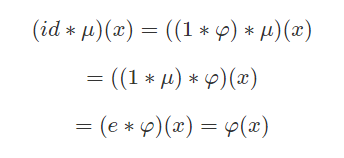Black Moonrise 题解

2019-05-07 17:08:19

## Black Moonrise 题解

### 算法4

$$ans=\sum_{i=1}^{\infty} xF(x)$$

$$G(x)=\sum_{x|d}F(d)$$

$$F(x)=\sum_{x|d}\mu(\frac{d}{x})G(d)$$$$ans=\sum_{d=1}^\infty G(d)\varphi(d)$$

$$G(d)=(\sum_{i=l}^r[d|a_i])^2$$

• star
首页